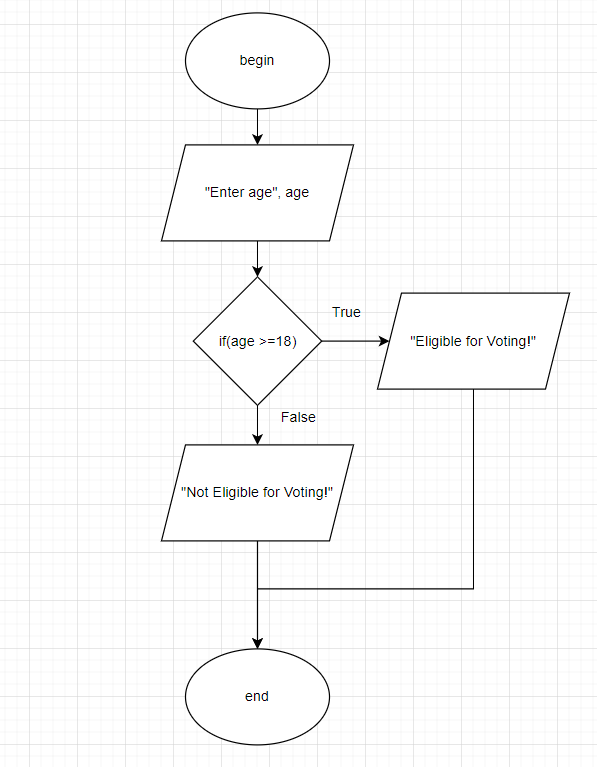# flowchart and pseudocode examples

### All Contributions

Pseudocode for Swapping Two Variables

In this pseudocode we will swap the values of two variables using a temporary variable. Here, Let’s learn how to swap values of two integer variables.

### Pseudocode Swap to Numbers with Entered by the User

swap two variables without taking value from user.

``````BEGIN

#define variables and assing values to variables
NUMBER num1=10, num2=15, temp

#assign num1 to temp
temp=num1

#assing num2 to num1
num1=num2

#assign temp to num2
num2=temp

OUTPUT "number 1:"+num1
OUTPUT "number 2:"+num2

END``````

FlowChart:Pseudocode to Claculate Area and Perimeter of Circle

In geometry, the area enclosed by a circle of radius r is πr2. Here the Greek letter π represents a constant, approximately equal to 3.14159, which is equal to the ratio of the circumference of any circle to its diameter.

The formulas for the perimeter P and area A of a circle are given by:

P = 2 π r

A = π r 2

Pseudocode :Area

``````BEGIN
NUMBER r, area
INPUT r
area=3.14*r*r
OUTPUT area
END``````

Pseudocode: Perimeter

``````BEGIN
NUMBER r, perimeter
INPUT r
perimeter=3.14*2*r
OUTPUT perimeter
END``````

Flowchart :AreaFlowchart: Perimeter### Pseudocode program to calculate Body Mass Index (BMI)

The Body Mass Index (BMI) is a quick way to assess your body size simply with your weight and height, regardless of your gender. Quickly calculate your BMI and find out which category you fall into. The Body Mass Index (BMI) is the only index validated by the World Health Organization to assess an individual’s build and therefore health risks. The BMI makes it possible to determine whether one is the situation of thinness, overweight or obesity for example. In this program we will calculate BMI. Body mass index (BMI) is a measure of body fat based on height and weight that applies to adult men and women.

### Pseudocode– How to Calculate Body Mass index (BMI)

Write a program that calculates and displays a person’s body mass index (bmi) in pseudocode.

``````BEGIN
NUMBER height , weight , bmi
DISPLAY "Enter person's information:"

DISPLAY "height in inches? "
INPUT height
DISPLAY "weight in pounds? "
INPUT weight

bmi = weight / (height*height) * 703

IF bmi < 18.5
DISPLAY "underweight"
ELSE IF bmi < 20
DISPLAY "normal"
ELSE IF bmi < 30
DISPLAY "overweight"
ELSE
DISPLAY "obese"
END IF

END``````

FlowChart– How to Calculate Body Mass index (BMI)draw flowchart and write pseudocode to input your age check whether you are eligible for voting or not

To check that a person is eligible for voting or not, we need to check whether person’s age is greater than or equal to 18. For this we are reading age in a variable a and checking the condition a>=18, if the condition is true, “person will be eligible for voting” else not.

#### Program Source Code : pseudocode to check eligibility for voting

Pseudocode:

``````BEGIN
NUMBER age
DISPLAY "Enter age"

IF age >= 18
DISPLAY "Eligible for Voting! "
ELSE
DISPLAY "NOT Eligible for Voting! "
END IF

END``````

flowchart:total contributions (4)